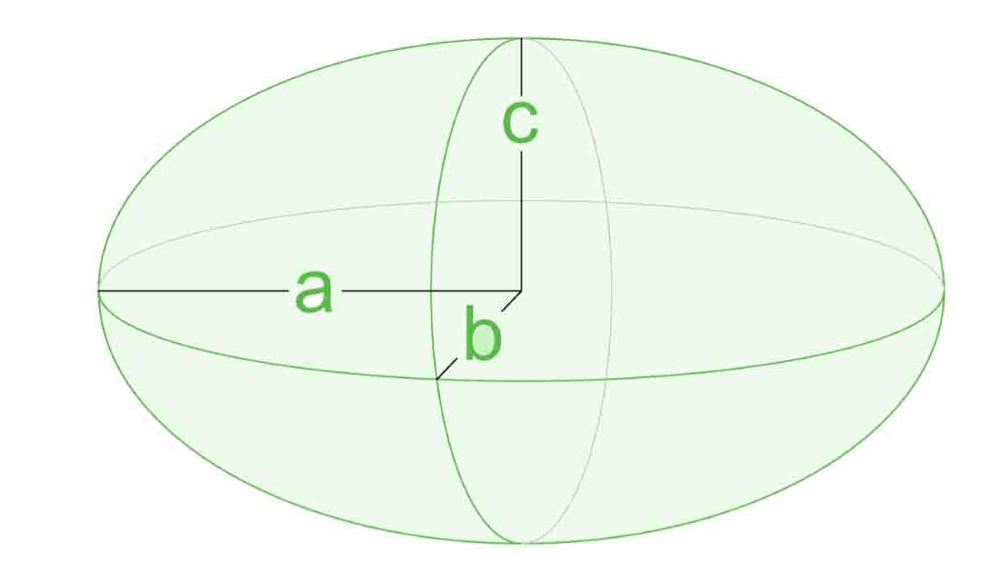# Program to calculate Surface Area of Ellipsoid

• Last Updated : 07 Aug, 2022

Given the length of the three semi-axes as A, B, and C, the task is to find the surface area of the given Ellipsoid.

Ellipsoid is a closed surface of which all plane cross-sections are either ellipses or circles. An ellipsoid is symmetrical about the three mutually perpendicular axes that intersect at the center. It is a three-dimensional, closed geometric shape, all planar sections of which are ellipses or circles.

An ellipsoid has three independent axes, and is usually specified by the lengths a, b, c of the three semi-axes. If an ellipsoid is made by rotating an ellipse about one of its axes, then two axes of the ellipsoid are the same, and it is called an ellipsoid of revolution, or spheroid. If the lengths of all three of its axes are the same, it is a sphere.Examples:

Input: A = 1, B = 1, C = 1
Output: 12.57

Input: A = 11, B = 12, C = 13
Output: 1807.89

Approach: The given problem can be solved by using the formula for Surface Area of the Ellipsoid as:Below is the implementation of the above approach:

## C++

 `// C++ program for the above approach` `#include ``#include ``#include ``using` `namespace` `std;` `// Function to find the surface area of``// the given Ellipsoid``void` `findArea(``double` `a, ``double` `b, ``double` `c)``{` `    ``// Formula to find surface area``    ``// of an Ellipsoid``    ``double` `area = 4 * 3.141592653``                  ``* ``pow``((``pow``(a * b, 1.6) + ``pow``(a * c, 1.6)``                         ``+ ``pow``(b * c, 1.6))``                            ``/ 3,``                        ``1 / 1.6);` `    ``// Print the area``    ``cout << fixed << setprecision(2)``         ``<< area;``}` `// Driver Code``int` `main()``{``    ``double` `A = 11, B = 12, C = 13;``    ``findArea(A, B, C);` `    ``return` `0;``}`

## Java

 `// Java program of the above approach``import` `java.util.*;` `class` `GFG{` `// Function to find the surface area of``// the given Ellipsoid``static` `void` `findArea(``double` `a, ``double` `b, ``double` `c)``{``    ` `    ``// Formula to find surface area``    ``// of an Ellipsoid``    ``double` `area = ``4` `* ``3.141592653` `* Math.pow((Math.pow(a * b, ``1.6``) +``                       ``Math.pow(a * c, ``1.6``) + Math.pow(b * c, ``1.6``)) /``                             ``3``, ``1` `/ ``1.6``);` `    ``// Print the area``    ``System.out.print(String.format(``"%.2f"``, area));``}` `// Driver Code``public` `static` `void` `main(String[] args)``{``    ``double` `A = ``11``, B = ``12``, C = ``13``;``    ` `    ``findArea(A, B, C);``}``}` `// This code is contributed by code_hunt`

## Python3

 `# Python3 program for the above approach``from` `math ``import` `pow` `# Function to find the surface area of``# the given Ellipsoid``def` `findArea(a, b, c):``    ` `    ``# Formula to find surface area``    ``# of an Ellipsoid``    ``area ``=` `(``4` `*` `3.141592653` `*` `pow``((``pow``(a ``*` `b, ``1.6``) ``+``            ``pow``(a ``*` `c, ``1.6``) ``+` `pow``(b ``*` `c, ``1.6``)) ``/` `3``, ``1` `/` `1.6``))` `    ``# Print the area``    ``print``(``"{:.2f}"``.``format``(``round``(area, ``2``)))` `# Driver Code``if` `__name__ ``=``=` `'__main__'``:``    ` `    ``A ``=` `11``    ``B ``=` `12``    ``C ``=` `13``    ` `    ``findArea(A, B, C)``    ` `# This code is contributed by SURENDRA_GANGWAR`

## C#

 `// C# program of the above approach``using` `System;` `class` `GFG{` `// Function to find the surface area of``// the given Ellipsoid``static` `void` `findArea(``double` `a, ``double` `b, ``double` `c)``{``    ` `    ``// Formula to find surface area``    ``// of an Ellipsoid``    ``double` `area = 4 * 3.141592653 * Math.Pow((Math.Pow(a * b, 1.6) +``                       ``Math.Pow(a * c, 1.6) + Math.Pow(b * c, 1.6)) /``                             ``3, 1 / 1.6);` `    ``// Print the area``    ``Console.Write(Math.Round(area, 2));``}` `// Driver Code``public` `static` `void` `Main(String[] args)``{``    ``double` `A = 11, B = 12, C = 13;``    ` `    ``findArea(A, B, C);``}``}` `// This code is contributed by shivanisinghss2110`

## Javascript

 ``

Output:

`1807.89`

Time Complexity: O(logn) as using inbuilt pow function
Auxiliary Space: O(1)

My Personal Notes arrow_drop_up